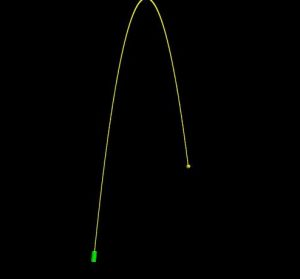# Cannonball in Visual Python

Just a very basic example of projectile motion in Visual Python. The cannon fires when the mouse is clicked in the window.```from visual import *

# Constants
g = 9.80            # (m/s^2)
v_0x = 5            # Initial speed in x-direction
v_0y = 50           # Initial speed in y-direction

# Set the display
scene = display(title='Cannonball',
x=0, y=0, width=700, height=700,
center=(0, 30, 0))

# Draw the cannon
location = [(0, 0, 0), (0.5, 5, 0)]
cannon = extrusion(pos=location, shape=outer-inner, color=color.green)

def position(t):
"""
Move the cannonball according to the equations of projectile motion.
"""
x = v_0x*t
y = v_0y * t - 0.5 * g * t ** 2
ball.pos = (x, y, 0)

# Shoot cannon when mouse is clicked and increment time
while True:
rate(1000)
if scene.mouse.clicked:
m = scene.mouse.getclick()
ball = sphere(pos=(0, 0, 0), radius=1, color=color.yellow, make_trail=True)
i = 0
flag = True
while flag:
rate(1000)
position(i)
i += 0.01
if ball.pos < 0:  # Stop before ball goes through the floor
flag = False
```

This site uses Akismet to reduce spam. Learn how your comment data is processed.